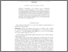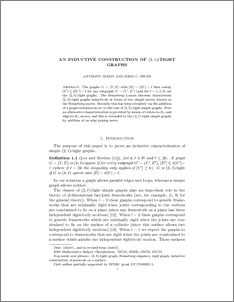# An inductive construction of (2,1)-tight graphs

Nixon, Anthony and Owen, John (2014) An inductive construction of (2,1)-tight graphs. Contributions to Discrete Mathematics, 9 (2). pp. 1-16. ISSN 1715-0868Preview
PDF (NixonOwen(2,1)Final)
NixonOwen_2_1_Final.pdf - Accepted Version

## Abstract

The graphs $G=(V,E)$ with $|E|=2|V|-\ell$ that satisfy $|E'|\leq 2|V'|-\ell$ for any subgraph $G'=(V',E')$ (and for $\ell=1,2,3$) are the $(2,\ell)$-tight graphs. The Henneberg--Laman theorem characterizes $(2,3)$-tight graphs inductively in terms of two simple moves, known as the Henneberg moves. Recently, this has been extended, via the addition of a graph extension move, to the case of $(2,2)$-tight simple graphs. Here an alternative characterization is provided by means of vertex-to-$K_4$ and edge-to-$K_3$ moves. This is extended to the $(2,1)$-tight simple graphs by the addition of an edge joining move.

Item Type:
Journal Article
Journal or Publication Title:
Contributions to Discrete Mathematics
Uncontrolled Keywords:
/dk/atira/pure/subjectarea/asjc/2600/2607
Subjects:
Departments:
ID Code:
73134
Deposited By:
Deposited On:
03 Mar 2015 11:04
Refereed?:
Yes
Published?:
Published